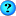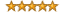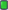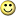ExetoolsSome help needed
 Register Forum Rules FAQ Calendar Mark Forums Read

 Notices https://forum.exetools.com This is the ONLY ONE domain that we use. Follow @exetools on Twitter and send me a message, I will choose whether to send the invitation code. Any password problems please mailto: 883600(at)qq(dot)com

#1
 Lana Posts: n/aSome help needed

I want to modify the pe table section of executable, the thing is that I need to change the 'raw size' value by adding 200 to it. The original Raw size value is 000001E4 and i want to know how to figure out the new value after adding 200 to it. Im sorry I really dont know much about this sort of thing. thanks in advance!#2
 karlss0n Posts: n/a
aligment of exe is 8 bit, so u can feel free to add 200h bytes to that segment
#3
 arkanoid Posts: n/a
and don't forget to change virtual size, if the fixed raw size is greater than virtual size. (unless they are padded with 0)
in such a case
if the section you want to modify is the last section you need to change the size of image too.
if it's the section in the middle, it's going to be little complex...
#4
 Lana Posts: n/a
hmm

hmmmm, karlss0n, thanks for the response but im still a bit confused....
I want to do this exactly;

000001E4 + 200 = 00000???
the "correct" answer is 000002C0, What I need to know how does this '000002C0' comes about. Where does that 'C" come from? lol, I'm sorry, I know this is probally a big stupid question and all.

-lana
#5
 JMILeaderJoin Date: Jan 2002 Posts: 1,627 Rept. Given: 5 Rept. Rcvd 199 Times in 99 Posts Thanks Given: 0 Thanks Rcvd at 96 Times in 94 PostsIt refers to the base-16 number system, which consists of 16 unique symbols: the numbers 0 to 9 and the letters A to F. The numbers are:

0, 1, 2, 3, 4, 5, 6, 7, 8, 9, A, B, C, D, E, F

For example, the decimal number 15 is represented as F in the hexadecimal numbering system. The hexadecimal system is useful because it can represent every byte (8 bits) as two consecutive hexadecimal digits. It is easier for humans to read hexadecimal numbers than binary numbers.
To convert a value from hexadecimal to binary, you merely translate each hexadecimal digit into its 4-bit binary equivalent. Hexadecimal numbers have either an 0x prefix or an h suffix. For example, the hexadecimal number

0x3F7A

translates to the following binary number:

0011 1111 0111 1010

The base 16 notational system for representing real numbers. The digits used to represent numbers using hexadecimal notation are 0, 1, 2, 3, 4, 5, 6, 7, 8, 9, A, B, C, D, E, and F. The following table gives the hexadecimal equivalents of the first few decimal numbers.

1 = 1 11 = B 21 = 15
2 = 2 12 = C 22 = 16
3 = 3 13 = D 23 = 17
4 = 4 14 = E 24 = 18
5 = 5 15 = F 25 = 19
6 = 6 16 = 10 26 = 1A
7 = 7 17 = 11 27 = 1B
8 = 8 18 = 12 28 = 1C
9 = 9 19 = 13 29 = 1D
10 =A 20 = 14 30 = 1E

and so on and so on.......

The hexadecimal system is particularly important in computer programming, since four bits (each consisting of a one or zero) can be succinctly expressed using a single hexadecimal digit. Two hexadecimal digits represent numbers from 0 to 255, a common range used, for example, to specify colors. Thus, in the HTML language of the web, colors are specified using three pairs of hexadecimal digits RRGGBB, where RR is the amount of red, GG the amount of green, and BB the amount of blue.

In hexadecimal, numbers with increasing digits are called metadromes, those with nondecreasing digits are called plaindrones, those with nonincreasing digits are called nialpdromes, and those with decreasing digits are called katadromes.

There's a good start.

Regards,
__________________
JMI
#6
 Satyric0n Posts: n/a
I don't see any way to land at 2C0h as the correct answer for any form of 1E4+200...

1E4h + 200h = 3E4h.
1E4h + 200d = 2ACh.
1E4h + 200o = 264h.

Where did you get 2C0h from?
#7
 Lana Posts: n/aThank you guys for all the input, you guys rule!arkanoid, yes, Im padding it with 0s. Thanks.

Satyric0n, someone who knows a bit more than me told me 2C0h was correct and I took hes word for it, sorry about that. =)

JMI, that is exactly what I was searching for!... I just didnt know what topic to search under. Thank You!
I have a lot of reading up to do...thank you everyone!!!!!!!!!

 Thread ToolsShow Printable VersionEmail this Page Display ModesLinear ModeSwitch to Hybrid ModeSwitch to Threaded ModePosting Rules You may not post new threads You may not post replies You may not post attachments You may not edit your posts BB code is On Smilies are On [IMG] code is Off HTML code is Off Forum Rules

All times are GMT +8. The time now is 03:57.

 Aaron's homepage - TopAlways Your Best Friend: Aaron, JMI, ahmadmansoor, ZeNiX
( 1998 - 2022 )